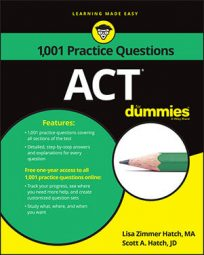##### ACTIt's very useful to know functions for the ACT Math exam. As you'll see in the following practice questions, function problems can be obvious, showing the trademark f(x), or they can be hidden in a word problem.

## Practice questions

1. A man threw a baseball from the top of a skyscraper at a height of 1,454 feet. The height of the baseball after the man threw it is a function of the time that has expired from the time he threw it. If t represents the time, in seconds, that has expired since the man threw the ball and h(t) represents the height, in feet, of the baseball, then I(t) = 1,454 – 16t2 + 8t + 4. What is the closest approximation, in feet, of the height of the baseball at 2 seconds?

A. 450 B. 727 C. 1,410 D. 1,454 E. 1,538

2. If

then f(g(20)) =

1. The correct answer is Choice (C). Consider this to be a function problem with h(t) as f(x). Functions are really just fancy substitution problems. To solve this function, simply substitute 2 for t on the right side of the equation, and then solve for h(t):

2. The correct answer is Choice (A).

Functions are really just fancy substitution problems. This one combines two functions, which means you have to perform two substitutions. Work from inside the parentheses out (that's easy because you're used to working this way under the standard order of operations).

The problem asks you to find f(g(20)). In other words, solve g(x) for g(20) in the second equation, and then plug what you get in for x in the other equation.

Substitute 20 for x in g(x):

Now substitute 3 for x in the equation for f(x):